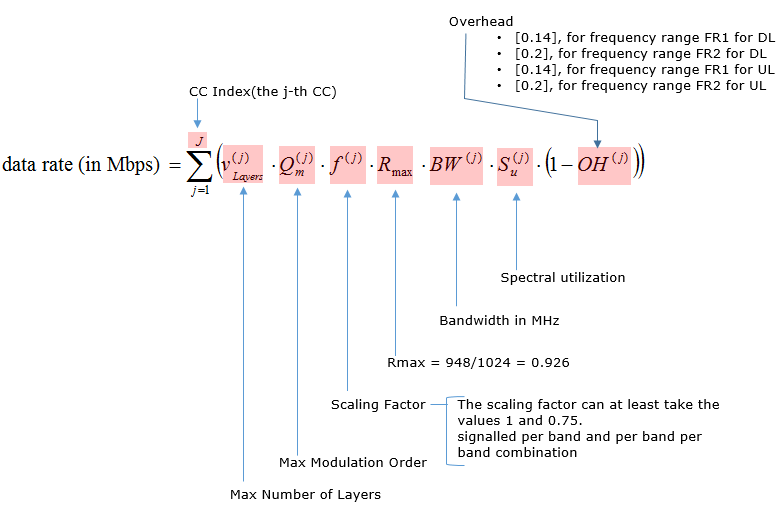## 5G Capacity & Throughput Calculation

Estimating the Maximum Throughput and 5G Capacity for modern Wireless Networks is complex and requires understanding of the 5G standards. This page is aimed at summarising what’s involved:

## 5G Maximum Capacity Estimation

Throughput estimation for 5G is complex, involving many factors and deep knowledge of the 5G standards. However, the rough estimation for a maximum throughput can roughly be estimated by following equation:

From:

<  38.306 – 4.1.2 Max data rate without ue-CategoryDL and ue-CategoryUL >5G Capacity:

The meaning of each parameter in this equation is as follows:5G Capacity Formula

## Explaining the formula in more detail:

For 5G NR, the approximate data rate for given number of aggregated carriers in a band or band combination is calculated using the above equation or formula. The following fields are used in 5G NR throughput calculation:

➤J : number of aggregated component carriers in a band or band combination
➤Rmax : 948/1024
• For the j-th CC, Vlayers(j) is the maximum number of layers
➤Qm(j) : Maximum modulation order, Qm is 2 for QPSK, 4 for 16QAM, 6 for 32QAM, 8 for 256QAM
➤f(j) : Scaling factor, can take any value from 1/0.8/0.75/0.4
➤μ : 5G NR Numerology, can take any value from 0 to 5.
➤Tsμ : Average OFDM symbol duration in a subframe for μ value,
•  Tsμ = 10-3/(14*2μ).
➤NPRBBW(j),μ : Maximum RB Allocation in bandwidth, BW(j)with numerology (μ),
BW(j) is UE supported maximum Bandwidth in given band or in band combinations.
REs are grouped into PRBs (Physical Resource Blocks). Each PRB consists of 12 Subcarriers.
➤OH(j) : Overhead which takes any of the following values.
• [0.14] → Frequency Range FR1 for DL
• [0.18] → Frequency Range FR2 for DL
• [0.08] → Frequency Range FR1 for UL
• [0.10] → Frequency Range FR2 for DL

Above mentioned formula has been used along with 5G NR Physical layer parameters and other 5G NR system parameters in order to develop 5G NR throughput calculator. One can refer following pdf which covers snapshot of 3GPP TS 38.306 document for more information on 5G NR data rate calculation. The maximum transmission bandwidth configuration NRB for each UE channel bandwidth and subcarrier spacing are specified in the tables below.

## 3GPP References

• 3GPP TS 38.306 V15.2.0 (2018-06)

## Maximum 5G Throughput & Capacity Calculators

We noted a few of examples of the maximum throughput calculators on the Internet as listed below.
Disclaimer: these are what we found on the Internet – results may vary and accuracy is not known or warranted.

## For Further Information

Please Contact Us